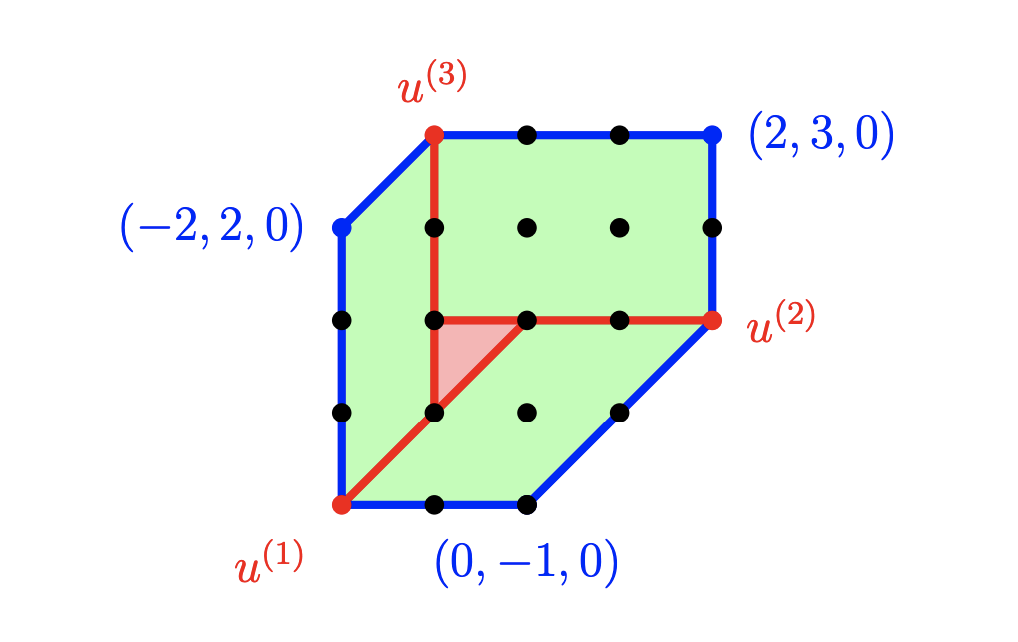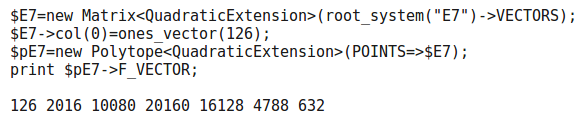# Problem 2

Consider the root system of type $$E_7$$. What is the f-vector of the convex hull of the roots? Same question for the zonotope they generate.

## Solution

Here we present two methods. First using Sage and second using Polymake:

### Sage

There is a simple example of computing f-vector at the begining

p = Polyhedron(vertices=[[1, 2, 3], [1, 3, 2],[2, 1, 3], [2, 3, 1], [3, 1, 2], [3, 2, 1], [0, 0, 0]])
p.f_vector()

e = RootSystem(['E',7])
E=e.ambient_space()
E1=E.roots()### Polymake

The following code will only work with Polymake version 3.2, due in October 2017

$E7=new Matrix<QuadraticExtension>(root_system("E7")->VECTORS);$E7->col(0)=ones_vector(126);
$pE7=new Polytope<QuadraticExtension>(POINTS=>$E7);
print \$pE7->F_VECTOR;